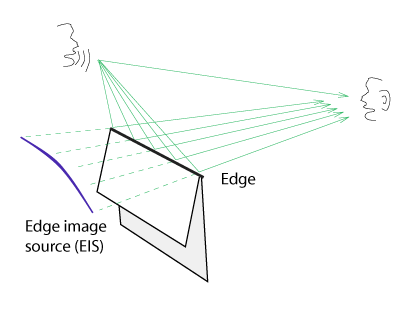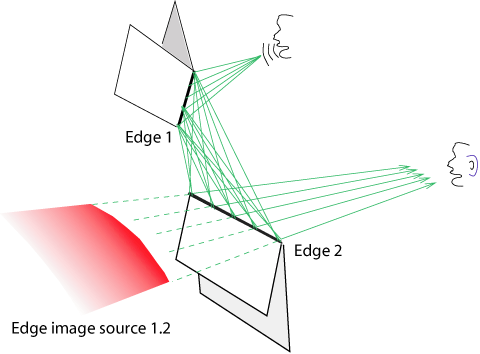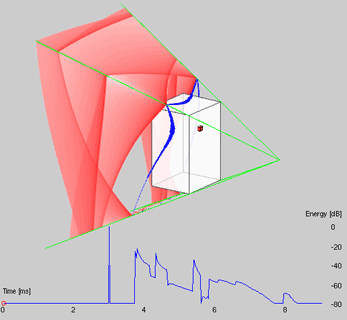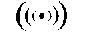# Visual Diffraction

### Ville Pulkki Laboratory of Acoustics and Audio Signal Processing Helsinki University of Technology

These pages show some visualizations of diffraction. Visualization is performed similarly as mirror image sources are visualized in the image-source method .

The image-source method is a method to simulate an acoustic space by replacing all surface reflections with image sources. This yields also an intuitive way to visualize acoustics. Some visualizations of basic cases are presented here.

The image-source method is valid only if there is no edges on surfaces, and if all angles between them are orthogonal. If a sound is reflected by a finite surface, there will occur diffraction in edges. Each point of an edge acts as a secondary source. Diffraction can be visualized analogous with the image source method. All diffractive edges are divided to small fractions, the direction from where the sound arrives to receiver is computed together with total sound propagation length respective to each fraction. Adjacent fractions are connected with a line. Diffraction thus forms a new type of image source, edge image source (EIS), which is illustrated by a curved line. For a single edge this is visualized as:A schematic visualization of first-order diffraction in one edge with one propagation path.

This figure has been drawn by hand, and the visualization is not as precise as it could be. Press here to see precise visualizations of first-order diffraction.

When the sound is diffracted two times on its propagation path from a source to a listener, an EIS of second order is formed:A schematic visualization of second-order diffraction.

Press here for precise visualizations of second-order diffraction.

This shows how the sound field of a loudspeaker decomposed to direct sound (small cube), first diffraction (lines) and second-order diffraction propagate to the listening position.Allen, J. B. and Berkley, D. A. (1979). "Image method for efficiently simulating small-room acoustics." J. Acoust. Soc. of Am., 65, 943-950.

Real and Virtual acoustics research at TKKThis URL: http://www.acoustics.hut.fi/